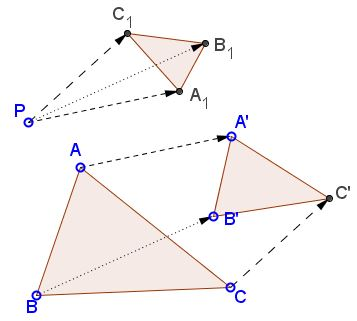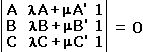# Directly Similar Figures What is that about? A Mathematical Droodle

Explanation### Explanation

The applet suggests the following statment:

Given two similar triangles ABC and A'B'C' and a point P, let A1 = P + AA', B1 = P + BB', and C1 = P + CC'. Then triangle A1B1C1 is similar to ABC and A'B'C'.The statement implies something more general, akin to the Fundamental Theorem of Directly Similar Figures and relates to the properties of spiral similarities. For any two directly similar figures S and S', if A is a generic point of S and A' is the corresponding point of S', then for any point P, P + AA' traces a figure similar two both S and S'.

The proof (in complex numbers) follows easily fromwith m = 1 and l = -1.

### References

1. I. M. Yaglom, Geometric Transformations II, MAA, 1968